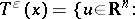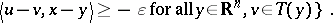# Proximal point methods in mathematical programming

The proximal point method for finding a zero of a maximal monotone operator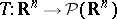generates a sequence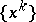, starting with any, whose iteration formula is given by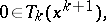(a1)

where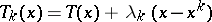and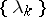is a bounded sequence of positive real numbers. The origin of the method can be traced back to the study of regularization of ill-posed problems (see [a3]; Regularization method; Ill-posed problems) and is closely related to Moreau–Yoshida convolution (see [a5]). A survey on the method can be found in [a4]. The basic properties of the method, as established in [a7], are as follows.

i) The sequenceis well defined (in the sense that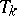has a unique zero), converges to a zero ofif such a zero exists, and is unbounded otherwise.

ii) Ifis strongly monotone, then the convergence rate ofis linear, and super-linear when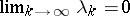. Whenis the subdifferential of a convex polyhedral function(i.e. the epigraph ofis a polyhedron), then convergence is finite.

iii) Convergence properties are preserved under inexact computation of the zero of, in the following sense: (a1) is replaced by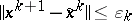, where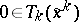and.

When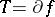(the subdifferential of a convex function), then (a1) is equivalent to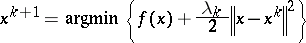(a2)

and the zeros ofare the minimizers of, so thatconverges to a minimizer of. Ifis the subdifferential of the dual objective of a convex optimization problem, then the sequencecoincides with the dual sequence generated by the augmented Lagrangian method (see [a6]).

All these properties hold also for maximal monotone operators in a Hilbert space, in which case convergence is understood in the sense of the weak topology.

The proximal point method can be applied to problems with convex constraints, e.g. the variational inequality problem, for a closed and convex set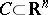, which consists of finding a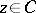such that there exists an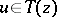satisfying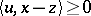for all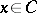. Whenwith convex,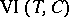reduces to minimizingsubject to. For applying the proximal point method toit suffices to replaceby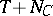, where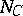is the normal cone operator of. In such a case, (a1) becomes equivalent to stating that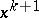solvesand (a2) becomes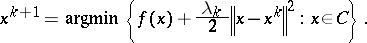There is another way to deal with constrained problems in the context of the proximal point method, without introducing the normal cone of, for the case whenhas non-empty interior and when a boundary-coercive Bregman functionwith zoneis available. In this case the method starts with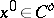(the interior of) and the iterative formula is still (a1) but now. In the optimization case, i.e., this formula is equivalent to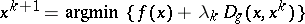where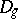is the Bregman distance associated with. This generalization of the proximal point method is an interior point algorithm, in the sense that the sequencelies in the interior of(cf. also Interior-point methods in mathematical programming). Under certain technical hypotheses on, satisfied, e.g., when, property i) above still holds, i.e. the sequenceconverges to a solution ofwhen a solution exists and is unbounded otherwise, [a8]. A property of this generalization, not shared by the original proximal point method (a1), is that for certain problems, including linear programming and quadratic programming, the limit of the sequenceis the solution of the problem which minimizes the Bregman distance to the initial iterate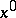(see [a2], [a10]). This procedure also generates augmented Lagrangian-type methods whenis the subdifferential of the dual objective of a convex optimization problem. For instance, whenis the non-negative orthant ofand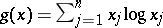, the sequencecoincides with the dual sequence of the Bertsekas exponential multipliers method (see [a1], [a11]).

Finally, some results are available for the proximal point method with Bregman distances under inexact solutions of the subproblems, in the spirit of property iii) above, [a9]. Convergence is guaranteed for the sequence generated by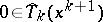, with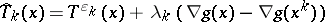, where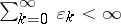and, for any monotone operatorand any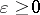,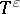is defined as